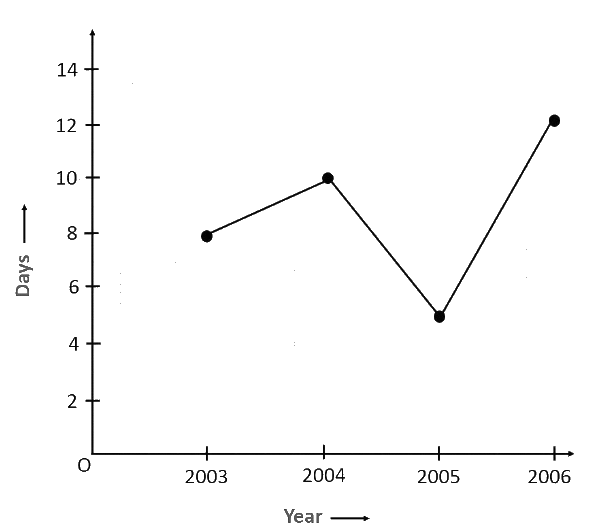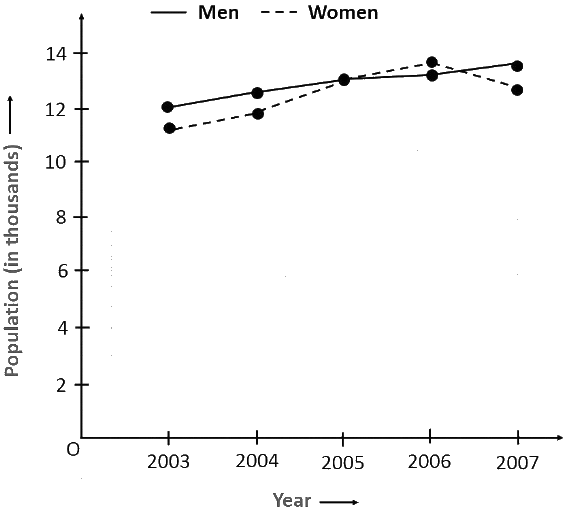# Ex.15.1 Q5 Introduction to Graphs Solutions - NCERT Maths Class 8

Go back to  'Ex.15.1'

## Question

Use the tables below to draw linear graphs.

(a) The number of days a hill side city received snow in different years.

 Year $$2003$$ $$2004$$ $$2005$$ $$2006$$ Days $$8$$ $$10$$ $$5$$ $$12$$

(b) Population (in thousands) of men and women in a village in different years.

 Year $$2003$$ $$2004$$ $$2005$$ $$2006$$ $$2007$$ Number of men $$12$$ $$12.5$$ $$13$$ $$13.2$$ $$13.5$$ Number of Women $$11.3$$ $$11.9$$ $$13$$ $$13.6$$ $$12.8$$

## Text Solution

Reasoning:

(a) Considering ‘Years’ on horizontal axis and ‘Days’ on vertical axis starting from origin i.e. ($$0,0$$).

(b) Considering ‘Years’ on horizontal axis and ‘Number of persons (i.e. ‘Men’ or ‘Women’) on vertical axis starting from origin i.e. ($$0,0$$). Represent ‘Number of men’ as a straight continuous line where as ‘Number of Women’ as a discontinuous straight line ( dashed line).

Steps:

(a) By taking the years on $$x$$-axis and the number of days on $$y$$-axis and taking scale as $$1$$ unit $$= 2$$ days on $$y$$-axis and $$2$$ unit $$= 1$$ year on $$x$$-axis, the linear graph of the given information can be drawn as follows.(b) By taking the years on $$x$$-axis and population on $$y$$-axis and scale as $$1$$ unit $$= 0.5$$ thousand on $$y$$-axis and $$2$$ unit $$=$$$$1$$ year on $$x$$-axis, the linear graph of the given information can be drawn as follows.Learn from the best math teachers and top your exams

• Live one on one classroom and doubt clearing
• Practice worksheets in and after class for conceptual clarity
• Personalized curriculum to keep up with school